4．约束条件为不等式的条件极值

y =f (x)x = (x1,x2,…,xn)

m个约束条件

gk(x)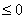k =1,2,…,m

[松弛变量法]  对每一约束不等式都引进一非负的松弛函数Si, 将它变为等式：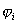=gi+Si=0

Si=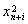y =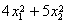x1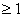约束条件可写为

g1=1- x1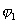=g1+S1=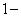x1+=0

y=4(1+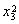)2+5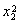D1=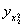=10>0

D2=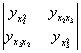=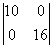=160>0

[拉格朗日乘数法]  引进松弛函数后，将约束不等式化为等式=gk+Sk(xn+k)=0,      k=1,,m

F=y+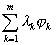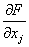=0      j=1,,(n+m)=0,    k=1,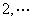,m

fI  (x1,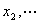,xn)=0,  i=1,,n